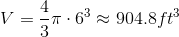# SSAT Upper Level Math : How to find the volume of a sphere

## Example Questions

### Example Question #2 : Volume Of A Sphere

A car dealership wants to fill a large spherical advertising ballon with helium. It can afford to buy 1,000 cubic yards of helium to fill this balloon. What is the greatest possible diameter of that balloon (nearest tenth of a yard)?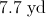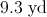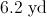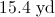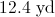Explanation:

The volume of a sphere, given its radius, is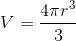Set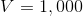, solve for, and double that to get the diameter.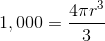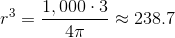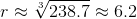The diameter is twice this, or 12.4 yards.

### Example Question #1 : Volume Of A Three Dimensional Figure

The diameter of a sphere is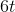. Give the volume of the sphere in terms of.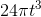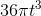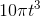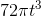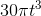Explanation:

The diameter of a sphere isso the radius of the sphere would be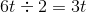The volume enclosed by a sphere is given by the formula: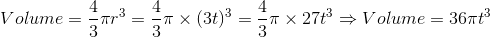### Example Question #2 : How To Find The Volume Of A Sphere

A spherical balloon has a diameter of 10 meters. Give the volume of the balloon.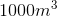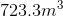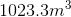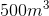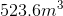Explanation:

The volume enclosed by a sphere is given by the formula: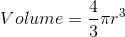whereis the radius of the sphere. The diameter of the balloon is 10 meters so the radius of the sphere would be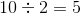meters. Now we can get: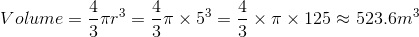### Example Question #3 : How To Find The Volume Of A Sphere

The volume of a sphere is 1000 cubic inches. What is the diameter of the sphere.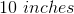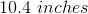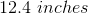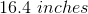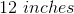Explanation:

The volume of a sphere is:Whereis the radius of the sphere. We know the volume and can solve the formula for: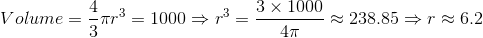inches

So we can get: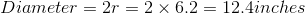### Example Question #4 : How To Find The Volume Of A Sphere

A sphere has a diameter ofinches. What is the volume of this sphere?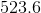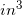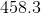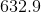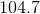Explanation:

To find the volume of a sphere, use the following formula: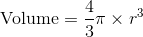, whereis the radius of the sphere.

Now, because we are given the diameter of the sphere, divide that value in half to find the radius.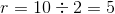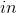Now, plug this value into the volume equation.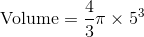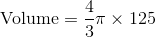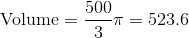### Example Question #5 : How To Find The Volume Of A Sphere

What is the volume of a sphere with a diameter of?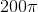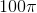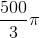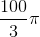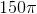Explanation:

Write the formula for the volume of the sphere.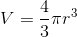The radius is half the diameter, which is five.  Substitute the value.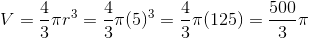### Example Question #2 : Volume Of A Three Dimensional Figure

What is the volume of a sphere with diameter 12 feet?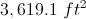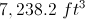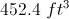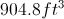None of the other answers are correctExplanation:

The radius of the sphere is half the diameter, or 6 feet; use the formula.

Setting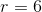: